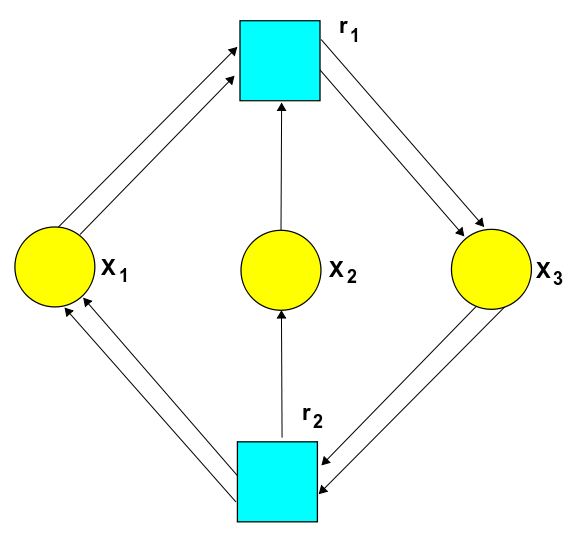# The Azimuth Project Experiments in bimolecular reactions (changes)

Showing changes from revision #3 to #4: Added | Removed | Changed

### A bimolecular reaction

For the general terms and definitions used in this project see

Here we will consider an example of a bimolecular reaction. This is example 6.3 in the paper

From Equation (12) page 13 we have the following chemical reaction network.

$k_1 : 2 X_1 + X_2 \rightarrow 2 X_3$
$k_2: 2 X_3 \rightarrow 2 X_1 + X_2$

We quote from the reference.

• when X1 = H2, X2 = O2, and X3 = H2O, the reversible creation of a molecule of water, when two molecules of the diatomic hydrogen gas combine with one molecule of the diatomic oxygen gas to produce two molecules of water. (The forward reaction produces energy, and the reverse reaction, breaking water to form hydrogen and oxygen, requires energy, for instance through electrolysis. The chemical reaction formalism used here does not account for energy production or consumption.)

### The stochastic Petri net### The rate equation

$\frac{d}{d t} X_1 = -2 k_1 X_1^2 X_2 + 2 k_2 X_3^2$
$\frac{d}{d t} X_2 = - k_1 X_1^2 X_2 + k_2 X_3^2$
$\frac{d}{d t} X_3 = 2 k_1 X_1^2 X_2 + -2 k_2 X_3^2$

### The master equation

$H = k_1(a_3^\dagger a_3^\dagger a_1 a_1 a_2 - a_1^\dagger a_1^\dagger a_1 a_1 a_2^\dagger a_2) + k_2 (a_1^\dagger a_1^\dagger a_2^\dagger -a_3^\dagger a_3^\dagger a_3 a_3)$

From this we arrive at the following

$z_3^2; 0 = k_1 c_1^2 c_2 - k_2 c_3^2$
$z_1^2 z_2; 0 = k_2 c_3^2 - k_1 c_1^2 c_2$

category: experiments